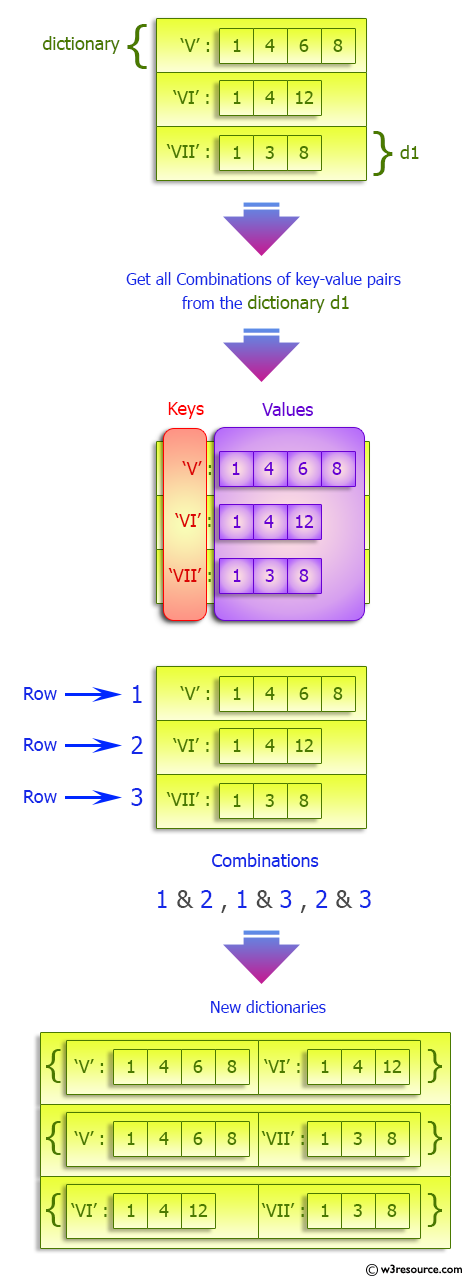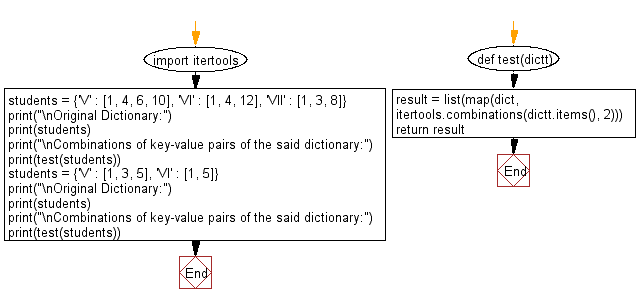﻿ Python: Combinations of key-value pairs in a given dictionary - w3resource# Python: Combinations of key-value pairs in a given dictionary

## Python dictionary: Exercise-58 with Solution

Write a Python program to get all combinations of key-value pairs in a given dictionary.

Sample Solution:

Python Code:

``````import itertools
def test(dictt):
result = list(map(dict, itertools.combinations(dictt.items(), 2)))
return result

students = {'V' : [1, 4, 6, 10], 'VI' : [1, 4, 12], 'VII' : [1, 3, 8]}
print("\nOriginal Dictionary:")
print(students)
print("\nCombinations of key-value pairs of the said dictionary:")
print(test(students))

students = {'V' : [1, 3, 5], 'VI' : [1, 5]}
print("\nOriginal Dictionary:")
print(students)
print("\nCombinations of key-value pairs of the said dictionary:")
print(test(students))
```
```

Sample Output:

```Original Dictionary:
{'V': [1, 4, 6, 10], 'VI': [1, 4, 12], 'VII': [1, 3, 8]}

Combinations of key-value pairs of the said dictionary:
[{'V': [1, 4, 6, 10], 'VI': [1, 4, 12]}, {'V': [1, 4, 6, 10], 'VII': [1, 3, 8]}, {'VI': [1, 4, 12], 'VII': [1, 3, 8]}]

Original Dictionary:
{'V': [1, 3, 5], 'VI': [1, 5]}

Combinations of key-value pairs of the said dictionary:
[{'V': [1, 3, 5], 'VI': [1, 5]}]
```

Pictorial Presentation:Flowchart:## Visualize Python code execution:

The following tool visualize what the computer is doing step-by-step as it executes the said program:

Python Code Editor:

Have another way to solve this solution? Contribute your code (and comments) through Disqus.

What is the difficulty level of this exercise?

Test your Programming skills with w3resource's quiz.

﻿

## Python: Tips of the Day

Invokes the provided function after ms milliseconds:

Example:

```from time import sleep

def tips_delay(fn, ms, *args):
sleep(ms / 1000)
return fn(*args)
print(tips_delay(
lambda x: print(x),
1000,
'w3r'
))
```

Output:

```w3r
None
```